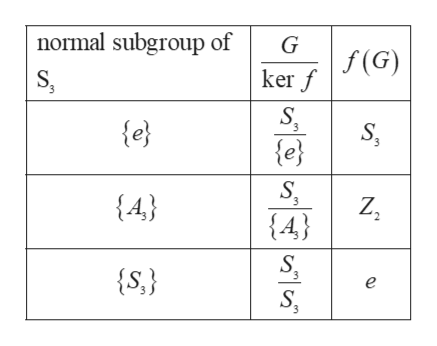Question
9 views
check_circle

Step 1

Let G and G’ are any groups and f is a homomorphism from G to G’ then

Step 2

Image and kernel of a group both are the subgroup of the group,

• The kernel of f, written ker f, is the set of elements g G such that f(g) = 1.
• The image of f, written im f, is the set of elements of H of the form f(g) for some g G.

Now if f is a homomorphism of a group G to G’ then the image of f is a subgroup of H, and the kernel of f is a normal subgroup of G.

Now let’s show that ker f is a normal subgroup of G. Given an element x ∈ G and an element g ∈ ker f , we have

Step 3

The normal subgroup of the S3 and co...help_outlineImage Transcriptionclosenormal subgroup of f(G) ker f S e} e} S Z2 {4} 3 {4} S e S fullscreen

### Want to see the full answer?

See Solution

#### Want to see this answer and more?

Solutions are written by subject experts who are available 24/7. Questions are typically answered within 1 hour.*

See Solution
*Response times may vary by subject and question.
Tagged in

### Math